# GCSE Maths Paper Two (Higher) Practice Paper in the Style of AQA - Calculator

In this assessment, students will be able to complete a timed GCSE Mathematics Paper 2 (Calculator) in the style of AQA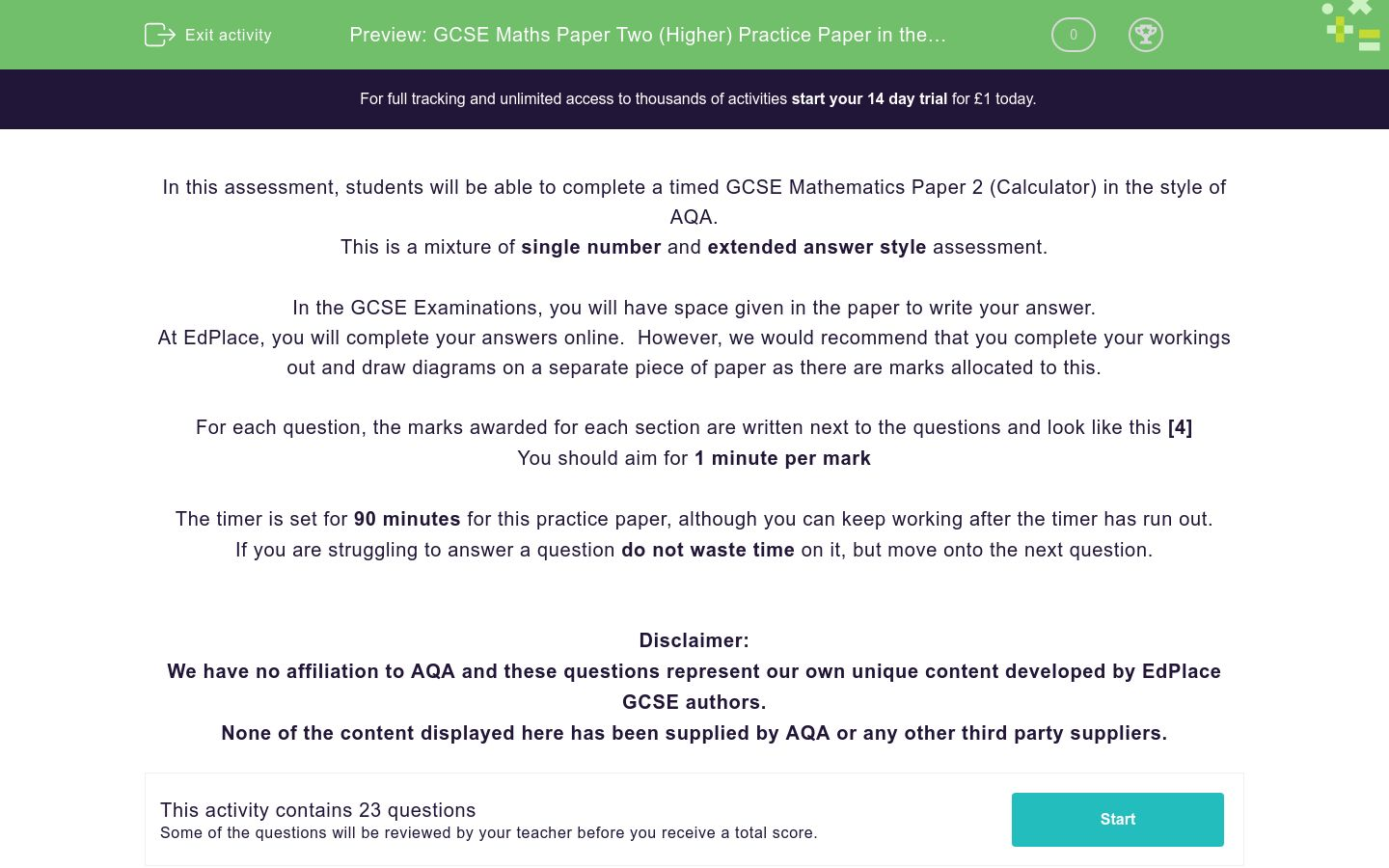Key stage:  KS 4

GCSE Subjects:   Maths

GCSE Boards:   AQA

Curriculum topic:   Higher Practice Papers

Curriculum subtopic:   Calculator Practice Papers

Difficulty level:### QUESTION 1 of 10

In this assessment, students will be able to complete a timed GCSE Mathematics Paper 2 (Calculator) in the style of AQA.

This is a mixture of single number and extended answer style assessment.

In the GCSE Examinations, you will have space given in the paper to write your answer.

At EdPlace, you will complete your answers online.  However, we would recommend that you complete your workings out and draw diagrams on a separate piece of paper as there are marks allocated to this.

For each question, the marks awarded for each section are written next to the questions and look like this 

You should aim for 1 minute per mark

The timer is set for 90 minutes for this practice paper, although you can keep working after the timer has run out.

If you are struggling to answer a question do not waste time on it, but move onto the next question.

Disclaimer:

We have no affiliation to AQA and these questions represent our own unique content developed by EdPlace GCSE authors.

None of the content displayed here has been supplied by AQA or any other third party suppliers.

This triangle is isosceles.Is the perimeter of this shape greater than 1 metre?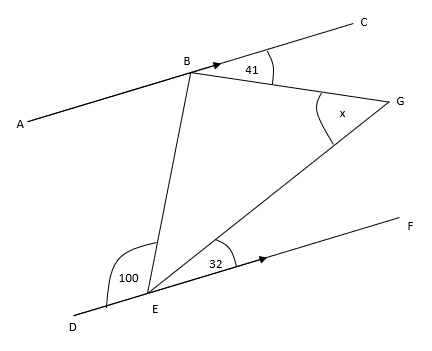ABC and DEF are parallel lines

Work out the size of angle x giving reasons for each stage of your working.



There are only red, black and blue marbles in a bag.

There are three times as many black marbles as red marbles and twice as many blue marbles as black.

Kim takes a marble from the bag at random.

Find the probability it is a black marble.



The diagram shows the side view of a step ladder.

The strut (BC) is one-quarter of the way up the ladder

The symmetrical cross-section shows two similar triangles.BC = 60 cm

Work out the vertical height of the ladder.



The volume of a brass instrument is 360 cm2

The instrument is made from copper and zinc.

Volume of copper: volume of zinc = 2:1

The density of copper is 8.96 g/cm3

The density of zinc is 7.13 g/cm3

Work out the mass of the instrument.



100 members of a triathlon club were asked if their favourite sport was cycling, swimming or running.

52 of the people asked were male

12 of the 41 who said cycling were female

19 men said running

17 people said swimming.

One of the female tri-athletes is picked at random, what is the probability she said swimming was her favourite sport?



Catherine puts £11000 in the bank in an account that pays compound interest for 3 years.

For the first two years, the bank pays X% interest.

After two years, she has £11669.90 in the bank.

As a reward for leaving her money untouched for two years, the interest for the third year is doubled.

How much money will Catherine have at the end of the three years?



The vectors a and b are shown on the grid.(a) Draw the vector -2b on the grid.     

(b) Work out b – 2a as a column vector.     

At the cinema, you can buy the following snacks…

2 types of popcorn

5 different drinks

9 types of sweet

Andy is going to have one of the following to eat while watching his film.

A drink and popcorn

A drink and a pack of sweets

A drink, some popcorn and some sweets.

How many different combinations of snack could Andy choose from?



OAB is a sector of a circle with centre O and a radius of 5.2cmThe area of the sector is 9.9 cm2

Calculate the perimeter of the sector, giving your final answer to 3 significant figures.



Show thatsimplifies towhere a, b, c and d are integers to be found.



On the grid, shade the region that satisfied all these inequalities.

x < 6      x + y > 8    y < x + 3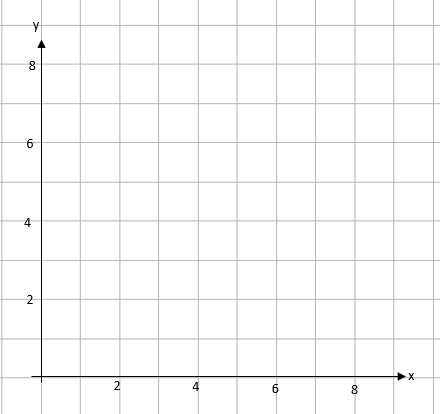James has to get a bus to work on Monday and Tuesday.

The probability he is late and misses the bus on Monday is 0.32.

If he misses the bus on Monday, the probability he will miss it on Tuesday is 0.22

If he catches the bus on Monday, the probability he will miss it on Tuesday is 0.04.

(a) Complete the probability tree diagram.(b) Find the probability he will catch the bus exactly once over the two days.          

ABC and ACD are triangles.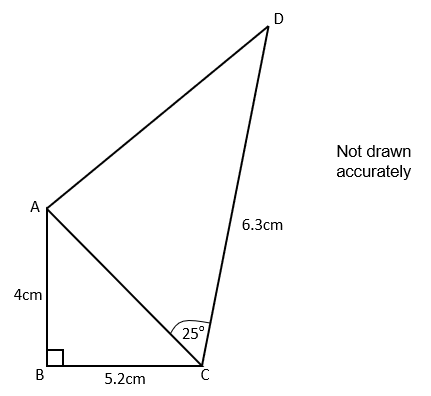Work out the area of ABCD.



The histogram shows information about the heights of some plants.(a) Complete the frequency table for this information.            

 Height (h cm) Frequency 24 ≤ h < 26 50 26 ≤ h < 27 27 ≤ h < 28 28 ≤ h < 30 30 ≤ h < 37

(b) Which group contains the median height of the plants?       

The straight line AB passes through the points (2,-1) and (5,-7)

The straight line CD has a gradient of 4 and passes through the origin.

Find the point where these two lines intersect.



Sarah is trying to find the density of a triangular prism made of metal.

She works out that…

The length of the shape is 15.1cm, correct to the nearest mm.

The cross-sectional area of the shape is 3.2cm2, correct to the nearest mm.

She weights the object and works out the mass as 16 g, correct to the nearest gram.

By considering the bounds, work out the density of the metal to a suitable degree of accuracy.



Avneet tries to solve this question        

Rationalise the denominator ofHer working is shown below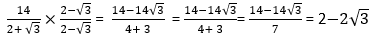Find these two mistakes.

The first 3 terms of a geometric sequence are given by(a) Find the value of x.      

(b) Show that the 5th term is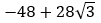A is (4,7) and B is (2,2).

Select the midpoint of AB.



(3,3)

(3,4.5)

(2,3)

(2,4.5)

The Bearing of A from B is 80°

What is the bearing of B from A?



80°

100°

180°

260°

What does AUB represent in the following probability statement P(AUB)?



A or B or both

A but not B

not A or B

A and B

Which of the following is a quadratic sequence?



2 5 10 17 26

3 5 7 9 11

1 1 2 3 5

2 4 8 16 32

• Question 1

This triangle is isosceles.Is the perimeter of this shape greater than 1 metre?



EDDIE SAYS• Question 2ABC and DEF are parallel lines

Work out the size of angle x giving reasons for each stage of your working.



EDDIE SAYS• Question 3

There are only red, black and blue marbles in a bag.

There are three times as many black marbles as red marbles and twice as many blue marbles as black.

Kim takes a marble from the bag at random.

Find the probability it is a black marble.



EDDIE SAYS• Question 4

The diagram shows the side view of a step ladder.

The strut (BC) is one-quarter of the way up the ladder

The symmetrical cross-section shows two similar triangles.BC = 60 cm

Work out the vertical height of the ladder.



EDDIE SAYS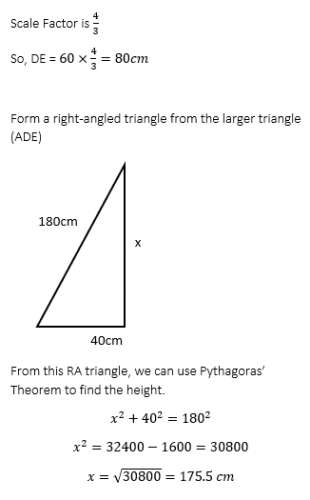• Question 5

The volume of a brass instrument is 360 cm2

The instrument is made from copper and zinc.

Volume of copper: volume of zinc = 2:1

The density of copper is 8.96 g/cm3

The density of zinc is 7.13 g/cm3

Work out the mass of the instrument.



EDDIE SAYS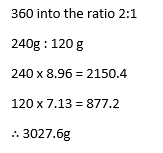• Question 6

100 members of a triathlon club were asked if their favourite sport was cycling, swimming or running.

52 of the people asked were male

12 of the 41 who said cycling were female

19 men said running

17 people said swimming.

One of the female tri-athletes is picked at random, what is the probability she said swimming was her favourite sport?



EDDIE SAYS• Question 7

Catherine puts £11000 in the bank in an account that pays compound interest for 3 years.

For the first two years, the bank pays X% interest.

After two years, she has £11669.90 in the bank.

As a reward for leaving her money untouched for two years, the interest for the third year is doubled.

How much money will Catherine have at the end of the three years?



EDDIE SAYS• Question 8

The vectors a and b are shown on the grid.(a) Draw the vector -2b on the grid.     

(b) Work out b – 2a as a column vector.     

EDDIE SAYS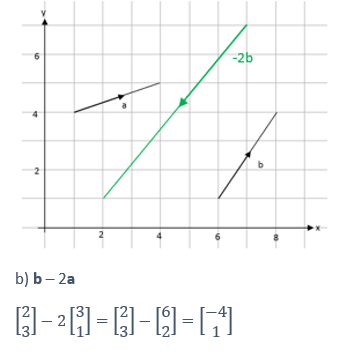• Question 9

At the cinema, you can buy the following snacks…

2 types of popcorn

5 different drinks

9 types of sweet

Andy is going to have one of the following to eat while watching his film.

A drink and popcorn

A drink and a pack of sweets

A drink, some popcorn and some sweets.

How many different combinations of snack could Andy choose from?



EDDIE SAYS• Question 10

OAB is a sector of a circle with centre O and a radius of 5.2cmThe area of the sector is 9.9 cm2

Calculate the perimeter of the sector, giving your final answer to 3 significant figures.



EDDIE SAYS• Question 11

Show thatsimplifies towhere a, b, c and d are integers to be found.



EDDIE SAYS• Question 12

On the grid, shade the region that satisfied all these inequalities.

x < 6      x + y > 8    y < x + 3EDDIE SAYS• Question 13

James has to get a bus to work on Monday and Tuesday.

The probability he is late and misses the bus on Monday is 0.32.

If he misses the bus on Monday, the probability he will miss it on Tuesday is 0.22

If he catches the bus on Monday, the probability he will miss it on Tuesday is 0.04.

(a) Complete the probability tree diagram.(b) Find the probability he will catch the bus exactly once over the two days.          

EDDIE SAYS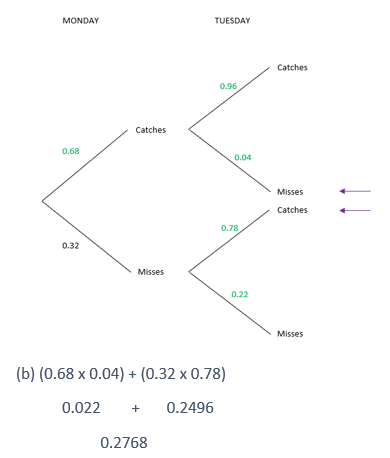• Question 14

ABC and ACD are triangles.Work out the area of ABCD.



EDDIE SAYS• Question 15

The histogram shows information about the heights of some plants.(a) Complete the frequency table for this information.            

 Height (h cm) Frequency 24 ≤ h < 26 50 26 ≤ h < 27 27 ≤ h < 28 28 ≤ h < 30 30 ≤ h < 37

(b) Which group contains the median height of the plants?       

EDDIE SAYS• Question 16

The straight line AB passes through the points (2,-1) and (5,-7)

The straight line CD has a gradient of 4 and passes through the origin.

Find the point where these two lines intersect.



EDDIE SAYS• Question 17

Sarah is trying to find the density of a triangular prism made of metal.

She works out that…

The length of the shape is 15.1cm, correct to the nearest mm.

The cross-sectional area of the shape is 3.2cm2, correct to the nearest mm.

She weights the object and works out the mass as 16 g, correct to the nearest gram.

By considering the bounds, work out the density of the metal to a suitable degree of accuracy.



EDDIE SAYS• Question 18

Avneet tries to solve this question        

Rationalise the denominator ofHer working is shown belowFind these two mistakes.

EDDIE SAYS• Question 19

The first 3 terms of a geometric sequence are given by(a) Find the value of x.      

(b) Show that the 5th term isEDDIE SAYS• Question 20

A is (4,7) and B is (2,2).

Select the midpoint of AB.



(3,4.5)
EDDIE SAYS
When you are finding the midpoint, the easiest way to do it is to add the x corrdinates together and half this. If you do the same with the y coordinates, you'll find the midpoint.
• Question 21

The Bearing of A from B is 80°

What is the bearing of B from A?



260°
EDDIE SAYS
The neat little trick to remember here is that reverse bearings always add to 360°.
• Question 22

What does AUB represent in the following probability statement P(AUB)?



A or B or both
EDDIE SAYS
Think of the Venn diagram, AUB means A OR B so it covers all the ones in both the circles.
• Question 23

Which of the following is a quadratic sequence?



2 5 10 17 26
EDDIE SAYS
Think what the word quadratic implies. It means there will be a square in there somewhere. The numbers in 2 5 10 17 26 are just one more than the square numbers, meaning this must be a quadratic sequence.